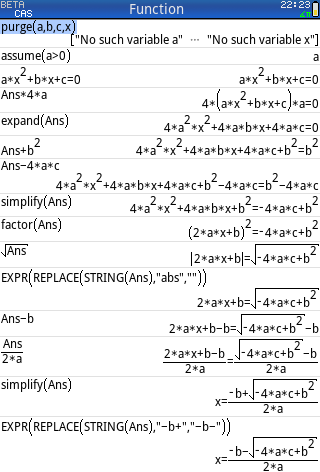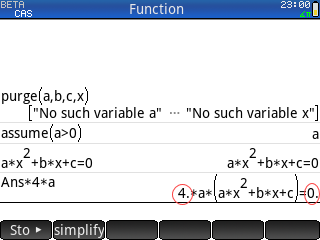Firmware 2021 - Scripts CAS on history view
04-17-2021, 04:02 AM (This post was last modified: 04-17-2021 04:14 AM by compsystems.)
Post: #1compsystemsSenior Member Posts: 1,329 Joined: Dec 2013
Firmware 2021 - Scripts CAS on history view
Hi, I'm having trouble executing a series of statements or scripts in CAS

Example:
Deduction of quadratic equations, step by step in CAS history view

A: First manually change to CAS mode, then CAS Settings SIMPLIFICATION TO NONE
[CAS]
[Shift]+[CAS]
simplify flag: None
Exact flag: ✔

B: Then run the following instructions

PHP Code:
purge(a,b,c,x); assume(a>0);  (a*x^2+b*x+c) = 0; Ans*4*a;      expand(Ans);    Ans+b^2;      Ans-4*a*c;    simplify(Ans); factor(Ans);   √(Ans);       expr(replace(string(Ans),"abs","")); Ans-b;          simplify(Ans);Ans/(2*a);    simplify(Ans);  expr(replace(string(Ans),"-b+","-b-"));

This is the expected result

PHP Code:
purge(a,b,c,x); [enter] "No such variable a",...assume(a>0); [enter] a(a*x^2+b*x+c) = 0; [enter] (a*x^2+b*x+c) = 0Ans*4*a;       [enter] ((a*x^2 +b*x +c) * 4*a) = 0expand(Ans);   [enter] (4*a^2*x^2 +4*a*b*x +4*a*c) = 0Ans+b^2;       [enter] (4*a^2*x^2 +4*a*b*x +4*a*c +b^2 ) = (b^2)Ans-4*a*c;     [enter] (4*a^2*x^2 +4*a*b*x +4*a*c +b^2 -4*a*c) = (b^2 -4*a*c)simplify(Ans); [enter] (4*a^2*x^2 +4*a*b*x +b^2) = (-4*a*c +b^2)  factor(Ans);   [enter] (2*a*x +b)^2) = (-4*a*c +b^2)√(Ans);        [enter] (abs(2*a*x +b)) = (√(-4*a*c +b^2))expr(replace(string(Ans),"abs","")); [enter] (2*a*x +b) = (√(-4*a*c +b^2))Ans-b;          [enter] (2*a*x +b -b) = (√(-4*a*c +b^2)-b)simplify(Ans);  [enter] (2*a*x) = (√(-4*a*c +b^2)-2*b)Ans/(2*a);      [enter] (2*a*x/(2*a)) = (-b+√(-4*a*c+b^2))/(2*a)simplify(Ans);  [enter] x = (-b+√(-4*a*c+b^2))/(2*a)expr(replace(string(Ans),"-b+","-b-")); [enter] x = (-b-√[b^2-4*a*c])/(2*a)but now sometimes expressions or numbers are floated ((4. *a^2*x^2 +4. *a*b*x +4. *a*c) = 0) and the symbolic calculation is lost, please tell me if the same thing happens to you too.